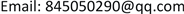1. 前言

2. 模型的建立2.1. 物理模型

1) 考虑到模拟中油的流动主要在变压器铁芯绕组外部与蒸发器之间，故暂忽略铁芯和高、低压绕组内部的导热问题。

2) 由于变压器流场呈对称分布，故取此变压器流场的1/2进行数值模拟，计算模型如图2所示。

3) 最外部的正方体模拟环境边界，尺寸为4000 mm × 4000 mm × 4000 mm。

2.2. 控制方程2.2.1. 连续方程

∂ ρ ∂ t + ∂ ( ρ u ) ∂ x + ∂ ( ρ v ) ∂ y + ∂ ( ρ w ) ∂ z = 0 (1)

2.2.2. 动量守恒方程

∂ ( ρ u ) ∂ t + d i v ( ρ u U ) = − ∂ p ∂ x + ∂ τ x x ∂ x + ∂ τ y x ∂ y + ∂ τ z x ∂ z + F x (2)

∂ ( ρ v ) ∂ t + d i v ( ρ v U ) = − ∂ p ∂ x + ∂ τ x y ∂ x + ∂ τ y y ∂ y + ∂ τ z y ∂ z + F y (3)

∂ ( ρ w ) ∂ t + d i v ( ρ w U ) = − ∂ p ∂ x + ∂ τ x z ∂ x + ∂ τ y z ∂ y + ∂ τ z z ∂ z + F z (4)

2.2.3. 能量守恒方程

∂ ( ρ T ) ∂ t + d i v ( ρ U T ) = d i v ( λ c p g r a d T ) + S T (5)

ρ = ρ 0 [ 1 − β ( T − T 0 ) ] (6)

ρ 0 为流体密度，kg/m3；T0为环境温度，K； β ——热膨胀系数，1/K；

2.3. 网格划分

Physical properties of transformer insulation oi

2.4. 边界条件与求解器设置

3. 数值模拟的结果3.1. 流场情况

3.2. 速度矢量

3.3. 温度分布

4. 结论

1) 本文通过模拟变压器油在地下变压器与蒸发换热器之间的流动，得到了其流场和温度场，这对地下变压器的冷却提供了一个新的改进思路和优化方案。

2) 顶层油温较高，速度也大，且在油箱顶部形成了旋涡，增强了换热。自然对流时在变压器和蒸发换热器的接口处以及铁芯绕组附近油流动的速度最快。

3) 通过对比不同位置蒸发换热器模型的流动和传热情况，发现蒸发换热器和变压器连接位置对变压器散热有一定的影响，相同工况下，蒸发换热器位置高的换热效果更好。